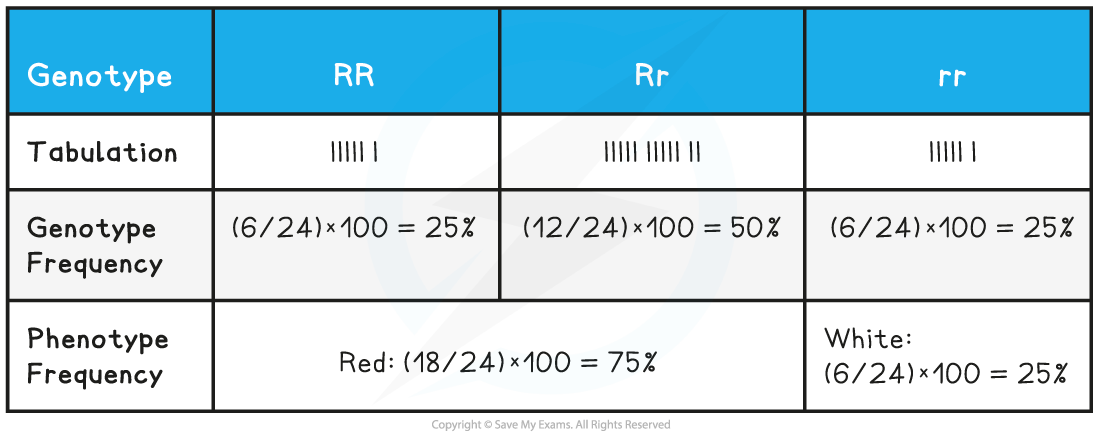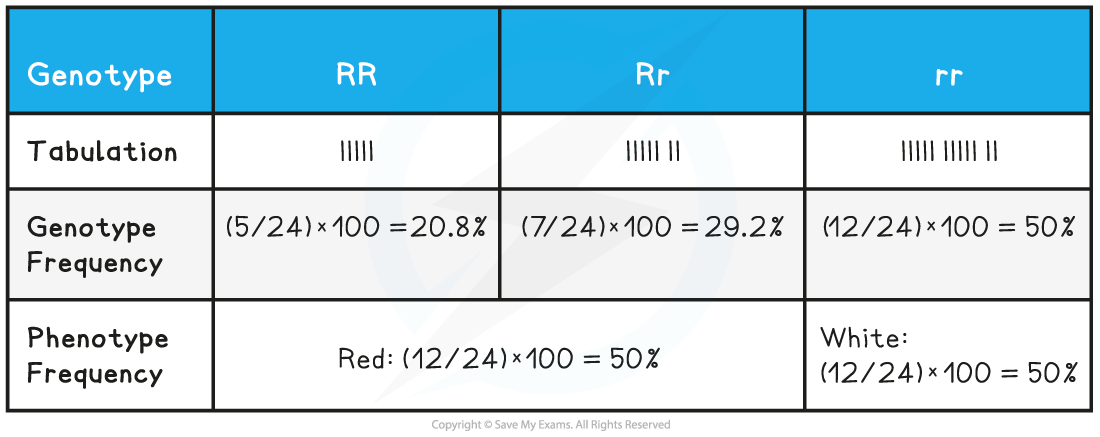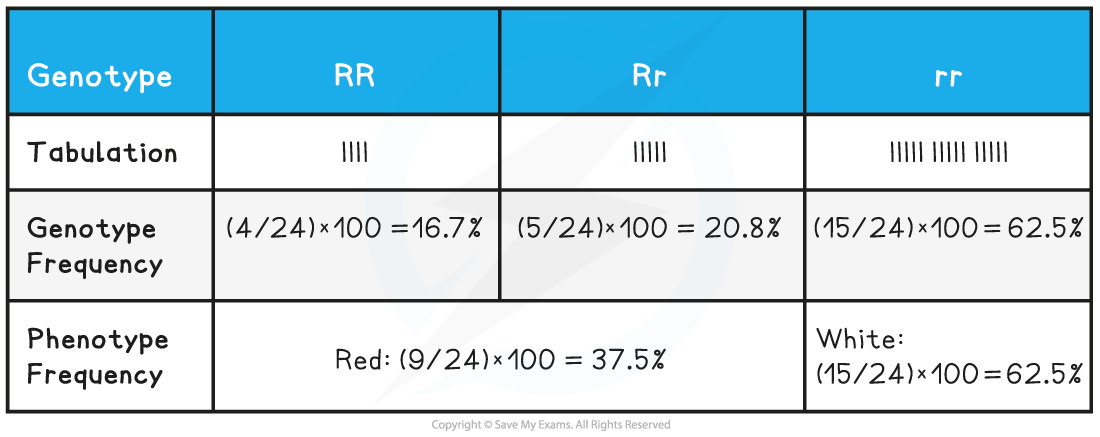# AQA A Level Biology复习笔记7.3.8 Investigating the Effects of Random Sampling on Allele Frequencies

### Practical Skill: Investigating the Effects of Random Sampling on Allele Frequencies

• Investigations to mimic the effects of random sampling on allele frequencies in a population can be devised using coloured beads, marbles or even sweets
• An example of one such investigation is described below

#### Apparatus

• 24 beads in one colour and 24 beads in a different colour
• Jar or container
• Paper
• Pencil
• Calculator

#### Method

• Place 24 red beads and 24 white beads in a container
• Red beads represent a dominant allele (R)
• White beads represent a recessive allele (r)

• Shake the container to ensure the beads are well mixed
• Carry out a random 'mating' by drawing out two beads without looking
• Each pair of beads drawn represents the fusing of a pair of sex cells containing either red or white alleles

• Carry out 24 of these random 'matings' and note down the genotype of each offspring
• Ensure you place the beads back into the container after each draw

• The theoretical offspring genotype ratio (also known as the expected genotype ratio) if thousands of draws are made and there is no genetic drift should be 1:2:1
• RR = 25% (chance of drawing two red beads in a row)
• rr = 25% (chance of drawing two white beads in a row)

• A table of these theoretical results (for 24 random 'matings') is shown below:#### Results

• However, the chances of drawing exactly six double red beads (RR), twelve red and white beads (Rr) and six double white beads (rr), as shown in the table above, are very small
• The effects of chance can mean that the offspring genotype ratio is likely to differ from the theoretical 1:2:1 offspring genotype ratio
• This change in the expected offspring genotype ratio that occurs in a small breeding population is known as genetic drift

• The table below shows some example results from 24 random '"matings"• If these results now represent the alleles in a new population, the ratio of red and white alleles are different from the 24 red alleles and 24 white alleles in the original population:
• Number of red alleles in new population = 5 x 2 (from RR) + 7 (from Rr) = 17
• Number of white alleles in new population = 12 x 2 (from rr) + 7 (from Rr) = 31

• As the white allele frequency has increased to 31 and the red allele frequency has decreased to 17, genetic drift has occurred
• If 24 more random 'matings' are now carried out from the new population (i.e. by placing 17 red beads and 31 white beads in the container), the effect of genetic drift on the allele frequencies in the population can become even greater
• The table below shows some example results from this second round of 24 random 'matings'
• Number of red alleles in new population = 4 x 2 (from RR) + 5 (from Rr) = 13
• Number of white alleles in new population = 15 x 2 (from rr) + 5 (from Rr) = 35

• As the white allele frequency has now further increased to 35 and the red allele frequency has now further decreased to 13, an even greater amount of genetic drift has occurred### Maths Skill: Sampling & Genetic Drift

• Using some simple maths, experiments like the one described above can be used to demonstrate genetic drift by calculating how allele frequencies change in a small population over multiple generations
• For example, it is possible to calculate how the percentage frequency of the alleles in the population changes between generations:
• In the first generation (original population) there were 24 red alleles and 24 white alleles. This means the percentage allele frequencies were:
• (24 ÷ 48) × 100 = 50% red alleles
• (24 ÷ 48) × 100 = 50% white alleles

• In the second generation (after the first round of 'matings') there were 17 red alleles and 31 white alleles. This means the percentage allele frequencies were:
• (17 ÷ 48) × 100 = 35.4% red alleles
• (31 ÷ 48) × 100 = 64.6% white alleles
• This means that in one generation the frequency of red alleles decreased by 14.6% and the frequency of white alleles increased by 14.6%

• In the third generation (after the second round of 'matings') there were 13 red alleles and 35 white alleles. This means the percentage allele frequencies were:
• (13 ÷ 48) × 100 = 27.1% red alleles
• (35 ÷ 48) × 100 = 72.9% white alleles
• This means that in two generations (i.e. compared to the starting population before any 'matings' were carried out) the frequency of red alleles decreased by 22.9% and the frequency of white alleles increased by 22.9%